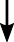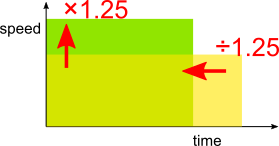#### You may also like### Mediant Madness

Kyle and his teacher disagree about his test score - who is right?### Tyneside Average Speed

Can you work out the average speed of the van?### Average Discovery

Find the missing number if the mean, median and mode are all the same.

# Marathon Mission

##### Age 14 to 16 Short Challenge Level:

Answer: 20%

Using inverse operations
100% + 25% = 1 + $\frac14$ = $\frac54$

speed      $\times$      time      = distance$\times \frac54$$\times$ ?$\times$ 1 because distance does not change
(new speed) $\times$ (new time) = distance

$\frac 54 \times$ ? = 1, so ? = $\frac45$ = 80%
80% of original time is a 20% reduction

Using a speed-time graphThe area on a speed-time graph represents distance.

Yellow rectangle - before 1 year training

Green rectangle - after 1 year trainingThe rectangles have the same area since the distance is still the same

Vertical scale factor $\times$ 125% = 1.25

So horizontal scale factor is the inverse

$\div$ 1.25 = $\div \frac54$ = $\times \frac45$ = $\times$ 80%

80% of original time is a 20% reduction

Using algebra
Before: speed $v$        time $\dfrac{26}{v}$

After:   speed $\frac54V$    time $\dfrac{26}{\frac54v}$

$=\dfrac{4\times26}{5v}$

$=\dfrac45\times\dfrac{26}v$

80% of original time is a 20% reduction

This problem is taken from the UKMT Mathematical Challenges.
You can find more short problems, arranged by curriculum topic, in our short problems collection.